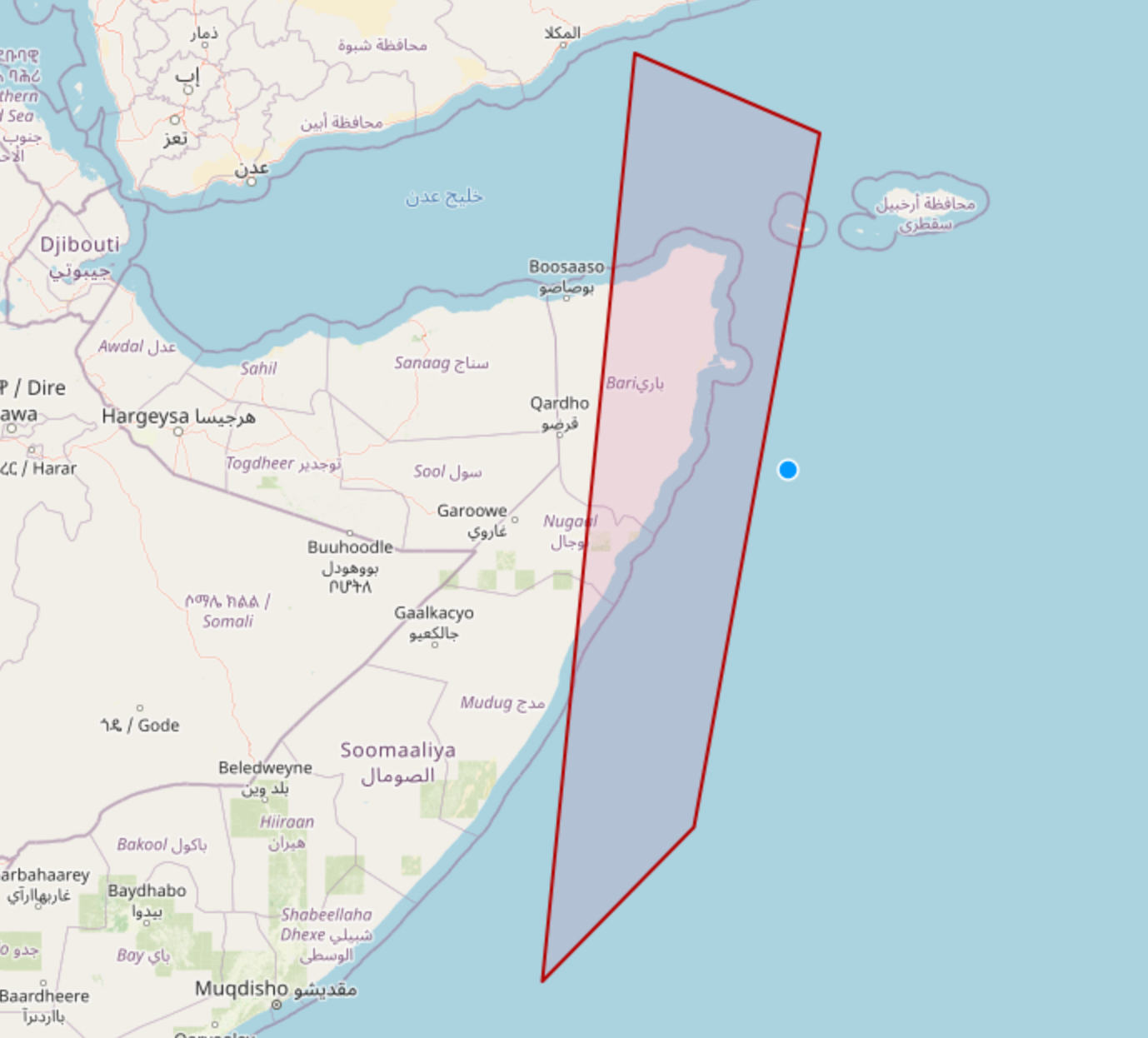# Python: Generate WKT from Lat Long Coordinates

Recently I’ve been playing around with geometry objects in WKT format while documenting Apache Pinot’s Geospatial functions. I then wanted to figure out how to generate a WKT string from a list of lat long coordinates, which we’ll learn how to do in this blog post.Figure 1. Python: Generate WKT from Lat Long Coordinates

We’re going to do all this using Python’s Shapely library, so let’s first install that library:

``pip install shapely``
 Note I learnt about the Shapely library from Davma’s StackOverflow question.

Let’s say we have a bunch of lat/long coordinates defined like this:

``````lat_longs = [
(50.854457, 4.377184),
(52.518172, 13.407759),
(50.072651, 14.435935),
(48.853033, 2.349553),
(50.854457, 4.377184)
]``````

We’re going to create a polygon based on these coordinates using the following code:

``````from shapely.geometry import Polygon
polygon = Polygon(lat_longs)``````

And getting a WKT string is as easy as calling the `wkt` property:

``print(polygon.wkt)``
Output
``POLYGON ((50.854457 4.377184, 52.518172 13.407759, 50.072651 14.435935, 48.853033 2.349553, 50.854457 4.377184))``

And finally, we can plot that polygon on a map using the OpenStreetMap WKT Playground.Figure 2. Polygon visualisation